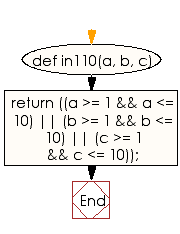﻿ Ruby Basic exercises: Check two integer values with in a range - w3resource# Ruby Basic Exercises: Check two integer values with in a range

## Ruby Basic: Exercise-9 with Solution

Write a Ruby program to check three numbers and return true if one or more of them are small. A number is called "small" if it is in the range 1..10 inclusive.

Ruby Code:

``````def in110(a, b, c)
return ((a >= 1 && a <= 10) || (b >= 1 && b <= 10) || (c >= 1 && c <= 10));
end
print in110(1, 12, 25),"\n"
print in110(10, 15, 33),"\n"
print in110(11, 25, 43),"\n"
``````

Output:

```true
true
false
```

Flowchart:Ruby Code Editor: